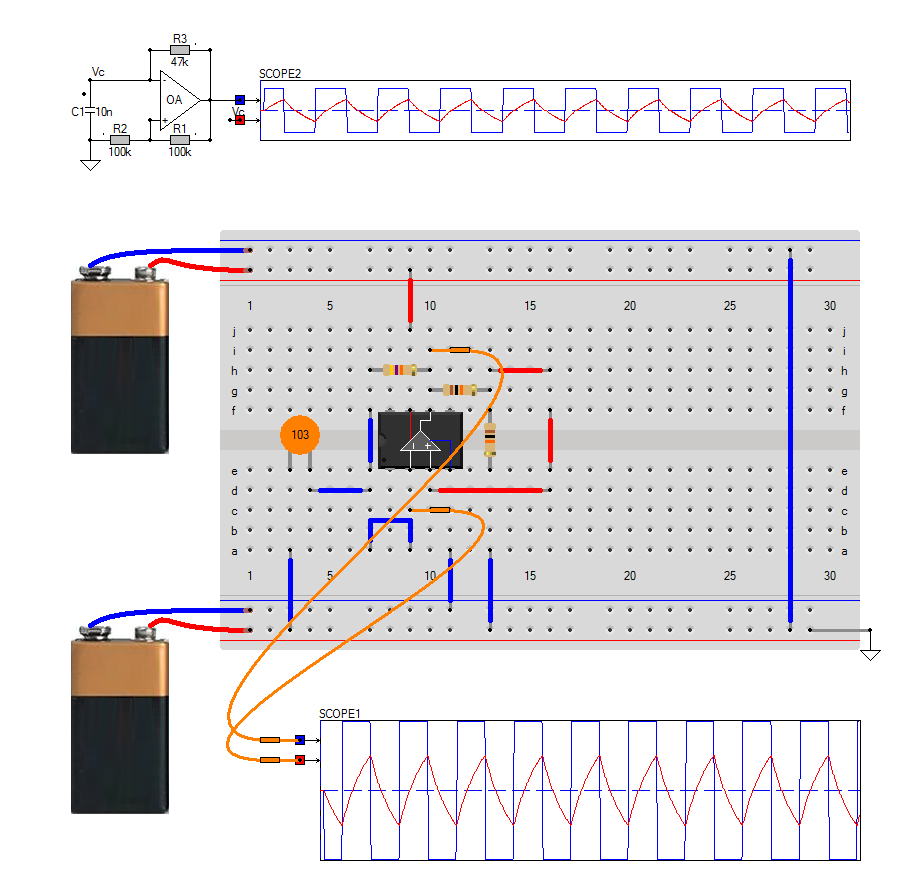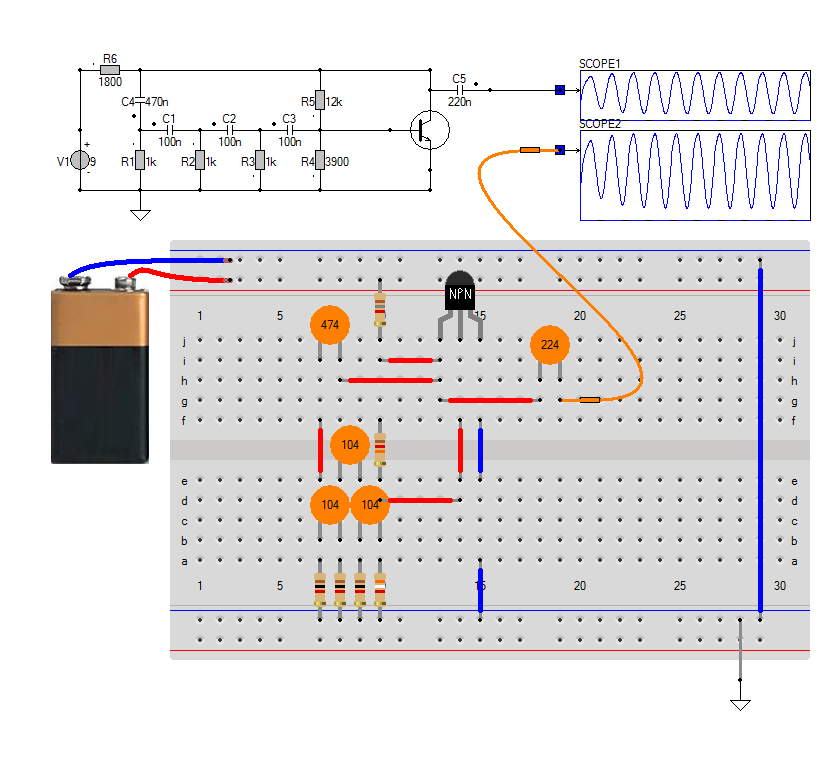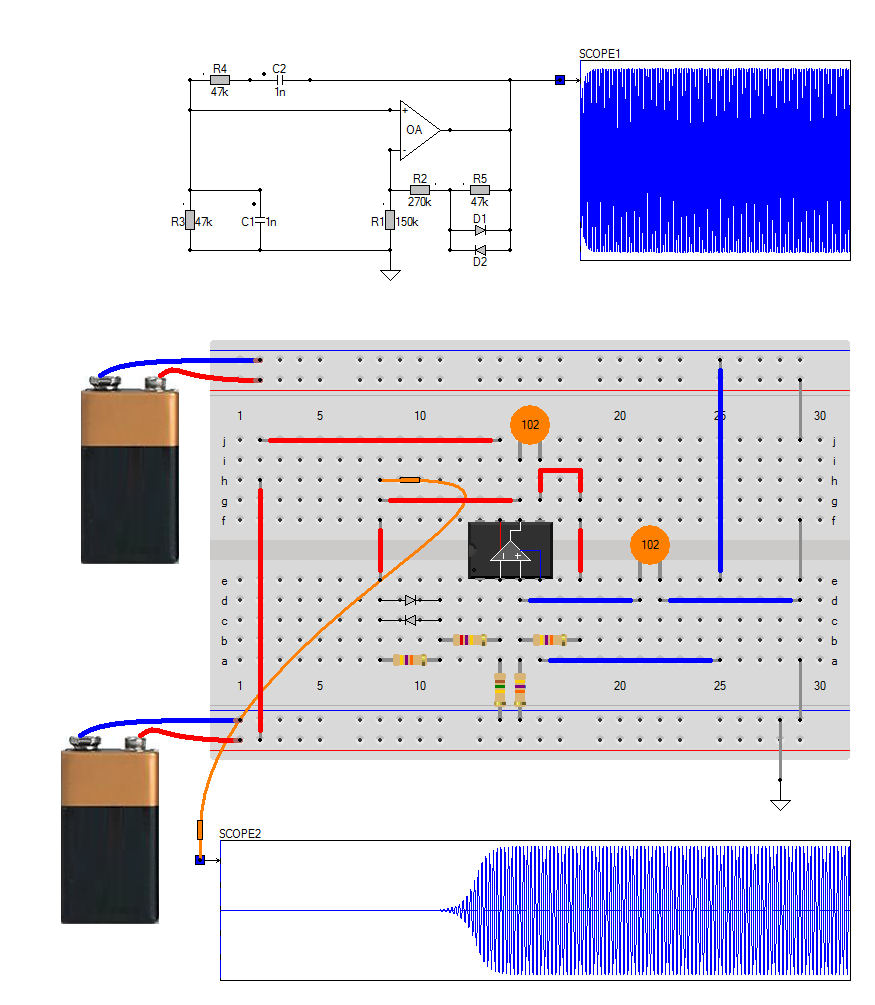## Oscillators

### Relaxation oscillator

Relaxation oscillators generate a changing voltage at a particular frequency by charging and discharging a capacitor through a resistor, and are often built around an operational amplifier.

To force the circuit to oscillate, we need automatically changing thresholds on the positive input pin: one �high� threshold which is above 0V, and another �low� threshold below 0V. This is known as hysteresis, and is the function of resistors R2 and R1

Instead of being tied to ground, the op amp�s positive input pin is connected to the junction of R1 and R2, which form a voltage divider between the op amp�s output and ground. In this case, since R1 and R2 are the same values, the voltage seen at the op amp�s positive input pin will mimic the voltage at the op amp�s output pin, only at half the voltage.

To get a better picture of how hysteresis and C1�s dis/charge time create an oscillation, let�s take a look at the positive and negative input pins of the op amp. The output of the opamp is shown in the scope as the blue trace. The square wave at the positive pin is exactly half of this signal. The red trace in the scope is the voltage over the capacitor C1

When the op amp�s output is at Vcc (high), the positive input pin is held at 1/2 Vcc by the R2/R1 resistor divider. At this point, C1 begins charging up through resistor R3 until it too crosses the $$\frac{Vcc}{2}$$ mark. The opamp sees that its inverting input is more positive than its non-inverting input, at which point it drives its output to Vss (low), since it�s an inverting amplifier.

Because the opamp�s positive input pin is controlled by the opamp�s output (specifically, it is set to half the voltage of the opamp�s output) the opamp�s positive input pin is now at half Vss, and C1 must discharge through resistor R3 until it crosses the $$\frac{Vcc}{2}$$ mark, at which point the opamp�s output will flip back to Vcc, beginning the cycle all over again.### Frequency calculation for the Relaxation oscillator

The RC time constant of C1 and R3 has an obvious contribution to the frequency of oscillation. Larger values of C1 and R3 will cause longer charge and discharge rates, thus producing lower frequency oscillations (smaller values will, of course, produce higher frequency oscillations).

But the hysteresis resistors R2 and R1 play a critical role here as well, because they control the voltage thresholds that the C1 needs to charge up to. If C1 only needs to charge and discharge to 1V and -1V respectively, it will obviously be able to do so faster than if it needed to charge and discharge to 10V and -10V.

To calculate the frequency of oscillation for any given combination of R1, R2, R3 and C1 (assuming Vcc and Vss are symmetric, e.g., +5V and -5V) you would have to take into account the symmetry of the voltage divider

If the hysteresis resistors R2 and R1 are equal to each other, the equations can be simplified $f = \frac{1}{2.2 \times R3 \times C1}$

We can predict the oscillation frequency for any given value of C1 and R3, and for our example

$F = \frac{1}{2.2 \times 47k\Omega \times10nF} = 1 kHz$

### Phase-shift Oscillator

For an oscillator to sustain oscillations indefinitely, sufficient feedback of the correct phase, that is �Positive Feedback� must be provided along with the transistor amplifier being used acting as an inverting stage to achieve this.

In an RC Oscillator circuit the input is shifted 180° through the amplifier stage and 180° again through a second inverting stage giving us �180° + 180° = 360°� of phase shift which is effectively the same as 0° thereby giving us the required positive feedback. In other words, the phase shift of the feedback loop should be �0°�.

The basic RC Oscillator which is also known as a Phase-shift Oscillator, produces a sine wave output signal using regenerative feedback obtained from the resistor-capacitor combination. This regenerative feedback from the RC network is due to the ability of the capacitor to store an electric charge, (similar to the LC tank circuit).

This resistor-capacitor feedback network can be connected as shown above to produce a leading phase shift (phase advance network) or interchanged to produce a lagging phase shift (phase retard network) the outcome is still the same as the sine wave oscillations only occur at the frequency at which the overall phase-shift is 360°.

By varying one or more of the resistors or capacitors in the phase-shift network, the frequency can be varied and generally this is done by keeping the resistors the same and using a 3-ganged variable capacitor.If all the resistors, R and the capacitors, C in the phase shift network are equal in value, then the frequency of oscillations produced by the RC oscillator is given as $f = \frac{1}{2 \pi \times R \times C \times \sqrt{2\times N}}$ where $$N$$ is the number of stages, being three in our example.

Since the resistor-capacitor combination in the RC Oscillator circuit also acts as an attenuator producing a total attenuation of less than $$\frac{1}{29}$$ across the three stages, the voltage gain of the amplifier must be sufficiently high enough to overcome these RC losses. Therefore, in our three stage RC network above, the amplifier gain must be equal too, or greater than, 29.

The loading effect of the amplifier on the feedback network has an effect on the frequency of oscillations and can cause the oscillator frequency to be up to 25% higher than calculated. Then the feedback network should be driven from a high impedance output source and fed into a low impedance load such as a common emitter transistor amplifier but better still is to use an Operational Amplifier as it satisfies these conditions perfectly.

### Wien-Bridge Oscillator

Of all the low-frequency oscillator configurations, the Wien bridge is the easiest to use. It is reliable, uses standard components, gives a good sine wave, and is fairly immune to the type of op amp around which it is designed.

A circuit maintains oscillation when the loop gain at the frequency of oscillation is unity, and the phase shift of the feedback signal at the frequency of oscillation is zero or a multiple of 2π.

First, the phase shift needs to be considered. In the Wien-Bridge, the series R and C produce a positive phase-shifted current with respect to the output voltage. When this phase-shifted current meets the parallel R and C, these latter components produce a negative phase-shifted voltage. At a specific frequency, the phase shift caused by the series R and C is offset by an equal and opposite phase shift caused by the parallel R and C, so the net phase shift is zero. When this occurs, the circuit is likely to oscillate.

To meet the requirements of oscillation (i.e., zero phase shift and unity gain), the op amp circuit needs to have a gain of 3 to overcome the attenuation resulting from the Wien-bridge network. From another perspective, to keep the two opamp inputs at the same voltage, the resistor network from the output to the inverting input needs to provide an attenuation of 3 to match the attenuation of the Wien-Bridge.

The gain in our example is calculated from the resistors in the feedback loop as $Gain= \frac{R1+R2+R5}{R1} = \frac{150k+270k+47k}{150k} = 3.1$

In theory, this technique is very convenient but it is not practical. Low tolerance resistors are easily included in a design. Finding accurate capacitors is, however, more difficult, and including capacitors with an accuracy greater than 20% can be costly.

A simple trick is to use two diodes in the feedback loop. The gain is now variable and depending on the output. In this example the gain of the non-inverting amplifier is controlled by the ratio of (R2+R5) to R1. Initially the gain set by R2+R5 and R1 will be just slightly greater than 3, this will allow oscillations to start.

Once the signal fed back from the output produces a waveform across R5 that approaches $$0.7 \cdot V_{pp}$$ the diodes will begin to conduct. Their forward resistance will reduce, and because they are in parallel with R5, this effectively reduces the value of R5 and so reduces the amplifier gain to. $Gain= \frac{R1+R2}{R1} = \frac{150k+270k}{150k} = 2.8$ making hte oscillation being stable.It may take a while before the oscillation starts, as there is no starting condition other than small leakage currents. Most opamps have a voltage and current offset at the input, and this will get the oscillator started!